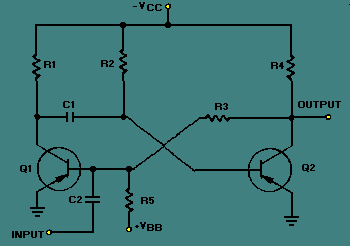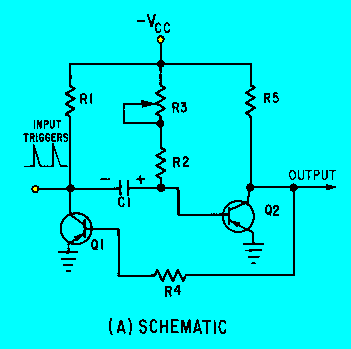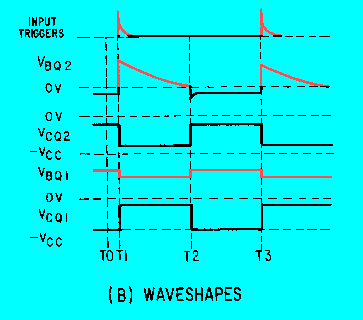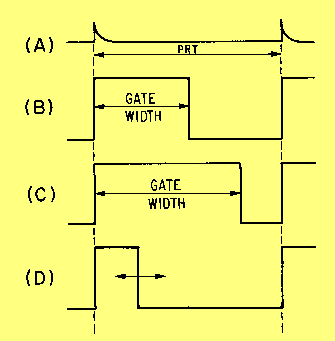transistor would initially go to cutoff because of circuit symmetry. ">Custom SearchFigure 3-10. - Monostable multivibrator block diagram.The schematic for a monostable multivibrator is shown in figure 3-11. Like the astable multivibrator, one transistor conducts and the other cuts off when the circuit is energized. Figure 3-11. - Monostable multivibrator schematic.Recall that when the astable multivibrator was first energized, it was impossible to predict which transistor would initially go to cutoff because of circuit symmetry. The one-shot circuit is not symmetrical like the astable multivibrator. Positive voltage VBB is applied through R5 to the base of Q1. This positive voltage causes Q1 to cut off. Transistor Q2 saturates because of the negative voltage applied from -VCC to its base through R2. Therefore, Q1 is cut off and Q2 is saturated before a trigger pulse is applied, as shown in figure 3-12. The circuit is shown in its stable state. Figure 3-12. - Monostable multivibrator (stable state).Let's take a more detailed look at the circuit conditions in this stable state (refer to figure 3-12). As stated above, Q1 is cut off, so no current flows through R1, and the collector of Q1 is at -VCC. Q2 is saturated and has practically no voltage drop across it, so its collector is essentially at 0 volts. R5 and R3 form a voltage divider from VBB to the ground potential at the collector of Q2. The tie point between these two resistors will be positive. Thus, the base of Q1 is held positive, ensuring that Q1 remains cutoff. Q2 will remain saturated because the base of Q2 is very slightly negative as a result of the voltage drop across R2. If the collector of Q1 is near -VCC and the base of Q2 is near ground, C1 must be charged to nearly VCC volts with the polarity shown. Now that all the components and voltages have been described for the stable state, let us see how the circuit operates (see figure 3-13). Assume that a negative pulse is applied at the input terminal. C2 couples this voltage change to the base of Q1 and starts Q1 conducting. Q1 quickly saturates, and its collector voltage immediately rises to ground potential. This sharp voltage increase is coupled through C1 to the base of Q2, causing Q2 to cut off; the collector voltage of Q2 immediately drops to VCC. The voltage divider formed by R5 and R3 then holds the base of Q1 negative, and Q1 is locked in saturation. Figure 3-13. - Monostable multivibrator (triggered).The one-shot multivibrator has now been turned on by applying a pulse at the input. It will turn itself off after a period of time. To see how it does this, look at figure 3-13 again. Q1 is held in saturation by the negative voltage applied through R3 to its base, so the circuit cannot be turned off here. Notice that the base of Q2 is connected to C1. The positive charge on C1 keeps Q2 cutoff. Remember that a positive voltage change (essentially a pulse) was coupled from the collector of Q1 when it began conducting to the base of Q2, placing Q2 in cutoff. When the collector of Q1 switches from -VCC volts to 0 volts, the charge on C1 acts like a battery with its negative terminal on the collector of Q1, and its positive terminal connected to the base of Q2. This voltage is what cuts off Q2. C1 will now begin to discharge through Q1 to ground, back through -VCC, through R2 to the other side of C1. The time required for C1 to discharge depends on the RC time constant of C1 and R2. Figure 3-14 is a timing diagram that shows the negative input pulse and the resultant waveforms that you would expect to see for this circuit description. Figure 3-14. - Waveforms of a monostable multivibrator (triggered).The only part of the operation not described so far is the short C1 charge time that occurs right after Q1 and Q2 return to their stable states. This is simply the time required for C1 to gain electrons on its left side. This charge time is determined by the R1C1 time constant. Another version of the monostable multivibrator is shown in figure 3-15. View (A) is the circuit and view (B) shows the associated waveforms. In its stable condition (T0), Q1 is cut off and Q2 is conducting. The input trigger (positive pulse at T1) is applied to the collector of Q1 and coupled by C1 to the base of Q2 causing Q2 to be cut off. The collector voltage of Q2 then goes -VCC. The more negative voltage at the collector of Q2 forward biases Q1 through R4. With the forward bias, Q1 conducts, and the collector voltage of Q1 goes to about 0 volts. C1 now discharges and keeps Q2 cut off. Q2 remains cut off until C1 discharges enough to allow Q2 to conduct again (T2). When Q2 conducts again, its collector voltage goes toward 0 volts and Q1 is cut off. The circuit returns to its quiescent state and has completed a cycle. The circuit remains in this stable state until the next trigger arrives (T3). Figure 3-15A. - Monostable miltivibrator and waveshapes. SchematicFigure 3-15B. - Monostable miltivibrator and waveshapes. WaveshapesNote that R3 is variable to allow adjustment of the gate width. Increasing R3 increases the discharge time for C1 which increases the cutoff time for Q2. Increasing the value of R3 widens the gate. To decrease the gate width, decrease the value of R3. Figure 3-16 shows the relationships between the trigger and the output signal. View (A) of the figure shows the input trigger; views (B) and (C) show the different gate widths made available by R3. Although the durations of the gates are different, the duration of the complete cycle remains the same as the pulse repetition time of the triggers. View (D) of the figure illustrates that the trailing edge of the positive alternation is variable. Figure 3-16. - Monostable multivibrator waveforms with a variable gate.The reason the monostable multivibrator is also called a one-shot multivibrator can easily be seen. For every trigger pulse applied to the multivibrator, a complete cycle, or a positive and negative alternation of the output, is completed. Q.5 In an astable multivibrator, which components determine the pulse repetition frequency?Q.6 What is another name for the monostable multivibrator?Integrated Publishing, Inc. - A (SDVOSB) Service Disabled Veteran Owned Small Business